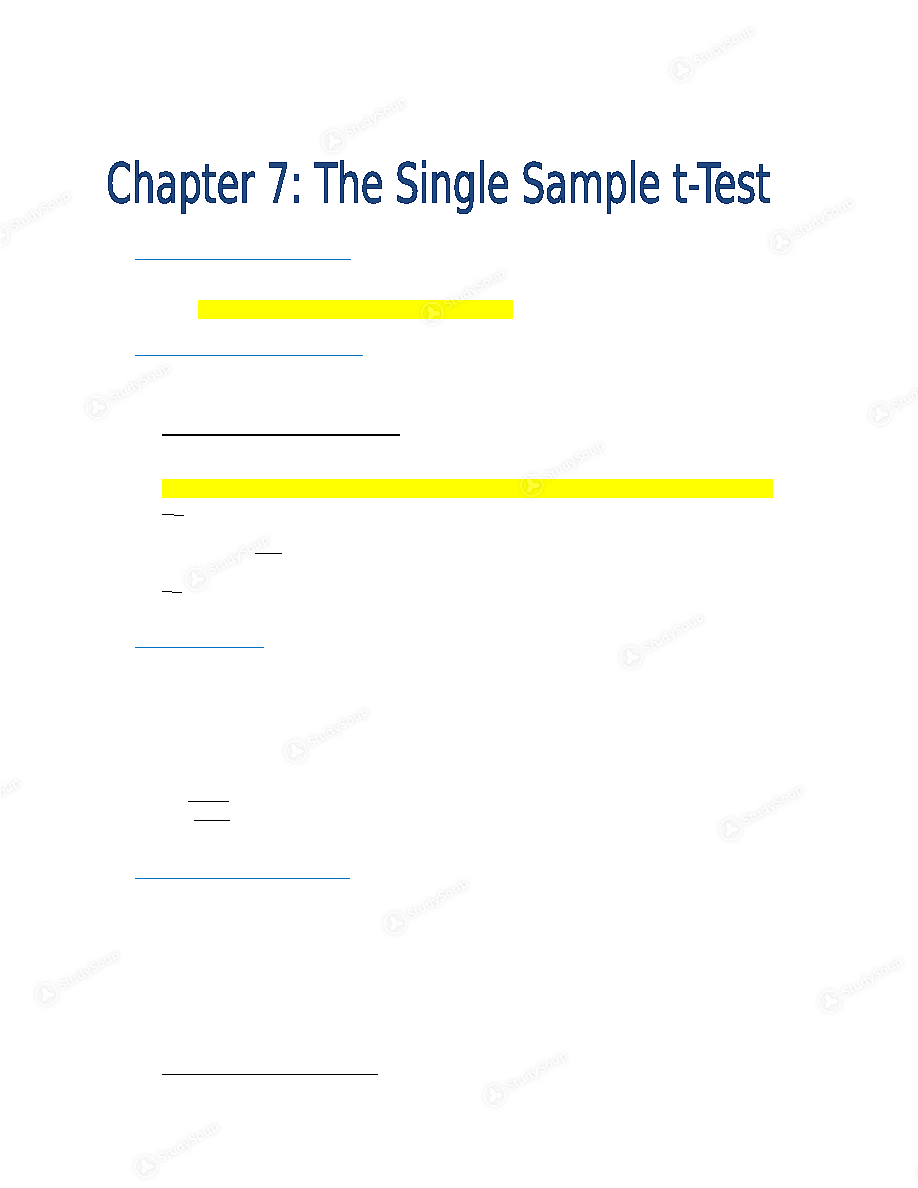×

# OleMiss - PSY 202 - Class Notes - Chapter 7- The Single Sample t-Test

### Created by: T'Keyah Jones Elite Notetaker

Schools > University of Mississippi > Psychology > PSY 202 > OleMiss - PSY 202 - Class Notes - Chapter 7- The Single Sample t-Test

OleMiss - PSY 202 - Class Notes - Chapter 7- The Single Sample t-Test

##### Description: These notes cover the beginning portion of The Single Sample t-Test.
This preview shows pages 1 - 2 of a 3 page document. to view the rest of the contentSeptember 29, 2016 a)       Single Sample t-Test Used to compare a sample mean to a population mean when the standard
deviation of the population is unknown
The steps are the same used for the z-test
b)       Going from “z” to “t” To compute a z-score, the population standard deviation (or variance) must
be known.
Estimated Standard Error
The sample deviation is substituted for the population standard deviation in
the calculation
Using this in the denominator of the test statistics led to the t-distribution
S
M Estimated standard error of means
Formula:    _S_
√N
M Standard error of means c)       t-Statistics Used to determine the number of standard deviations in a t-distribution that a
sample deviates from the mean value or differences stated in the null
The t-distribution is identical to the z-distribution Small sample sizes equal larger critical values t= M - μ
S
√N
d)       Degrees of Freedom Symbol: df
Formula: N-1
As the df of the sample variance increases, the shape of the t-distribution
changes
As the sample size increases, the sample variance more closely resembles
the population variance
To Know Critical Values1. Know the degree of freedom
2. Determine the type of tail (One-tailed or Two-tailed)
3. Know the alpha level
e)       Examples M= 62,000
N= 16
μ= 66,000
S= 12,000
Step 1: Determine Type of Test
Single sample t-test because we want to compare the mean from a sample
with an unknown standard deviation
Step 2: Assumptions
1. Random sample
2. Independent Observation
3. Normal distribution (dependent variable)
Step 3: Hypotheses
H
: μ FISH  ≥ 66,000 ; pollution does not have an effect H 1 : μ FISH  < 66,000 ; pollution does have an effect Step 4: Decision Rule
= .05
df= 15 (16-1)
Critical value: 1.753 (One-tail test on chart)
Step 5: Calculations
t= M - μ
t= 62,000 - 66,000 = -1.333         S_                                 12,000
√N                                   √16
Step 6: Interpret
We fail to reject the null because -1.333 is not in the tail of the distribution;
there is not enough evidence to suggest that the polluted lakes have
significantly fewer fish
Type II error
Example 2
N= 14
M= 42
S= 6.8
μ= 36
Step 1

This is the end of the preview. Please to view the rest of the contentJoin more than 18,000+ college students at University of Mississippi who use StudySoup to get ahead
3 Pages 42 Views 33 Unlocks
• Notes, Study Guides, Flashcards + More!Join more than 18,000+ college students at University of Mississippi who use StudySoup to get ahead